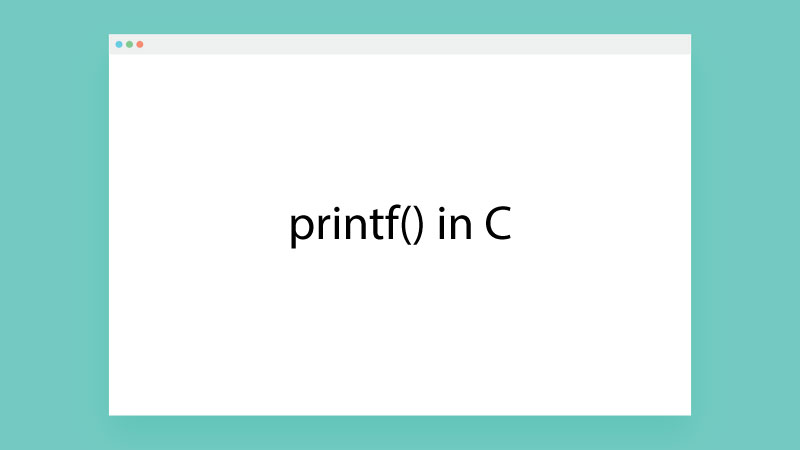# What is printf in C Programming

Janet | Checked On: 07 April 2023### Printf in C

printf() is a predefined inbuilt standard library function used to display output on the screen. The printf() function is defined in the stdio.h header file, printf() is case sensitive, it is always written as printf(). Generally printf() function looks like

In general, anything written in printf() function’s “ ” gets printed on the output screen.

#### printf() Example:

#include<stdio.h>

void main()

{

printf("Welcome to C Programming");

}

Output:

Welcome to C Programming

• printf() function is an inbuilt library function.
• printf() function is defined within C library’s “stdio.h” header file.
• To use printf() function, you must include the stdio.h header file in the C program.
• printf() function is case-sensitive i.e. printf() is different from Printf(), infact C is a case-sensitive programming language.

#### Use printf() Function in 2 Ways

For better understanding, the use of printf() function can be done in two ways.

• printf() function without variables/values
• printf() function with variables/values.

#### printf() function without variables/values

This scenario of printf() function is used to print simple/general messages as output in C programming, see below syntax and examples.

Syntax

##### Example

#include<stdio.h>

void main()

{

printf("This message from printf function \n");

printf("--------------------------------- \n");

printf("This is message 1 \n This is message 2 \n Both above two line are from same printf fuction\n");

printf("This is last message");

}

Output

This message from printf function

---------------------------------

This is message 1

This is message 2

Both above two line are from same printf fuction

This is last message

#### Newline Character (\n)

\n is used for new links in output. All text/content after \n will appear in the next line.

#### Placeholder

Placeholder is used to insert a value/variable value in a message in printf() function, the below table contains the type of value and placeholder representation.

Data Types [Placeholder Representation]

• int [%d]
• char [%c]
• float [%f]
• double [%lf]
• string [%s]
• octal [%o]

#### printf() function with variables/values

This scenario of printf() function is used to print a message along with some value stored in variables. We use placeholder representation to insert the value of the variable in the message. Let's understand with an example.

Syntax

printf(“Message with list of placeholder symbol”, variable1, variable2, variable3,....);

##### Example 1

#include<stdio.h>

void main()

{

int a=10;

int b=20;

int sum=a+b;

printf("Sum of %d and %d is : %d", a,b,sum);

}

Output

Sum of 10 and 20 is: 30

##### Rules/Explanation:

• In the above example a, b and sum are variables.
• All three %d is called a placeholder.
• %d symbols are replaced by int type values.
• variable order matters for example [ a,b,sum ---> 10,20,30 ] and [ a,sum,b ---> 10, 30, 20 ]
• Placeholder representation symbols and variable data type must match and the order should be correct.

##### Example 2

#include<stdio.h>

void main()

{

char name ='A';

int age =20;

float mark = 73.56;

printf("%c %d %f \n", name, age, mark);

printf("------------------------- \n");

printf(" Name=%c Age=%d Marks=%f \n", name, age, mark);

printf("------------------------- \n");

printf(" Name=%c \n Age=%d \n Marks=%f \n", name, age, mark);

}

Output

A 20 73.56

-------------------------

Name=A Age=20 Marks=73.56

-------------------------

Name=A

Age=20

Marks=73.56

Note: At the place of variables, you can directly pass values in printf() function. See the below example.

#include<stdio.h>

void main()

{

char name ='A';

float salary = 35800.75;

printf(" Mr. %c age is %d years and his salary is %f", name, 20, mark);

}

Output

Mr. A age is 20 years and his salary is 35800.75